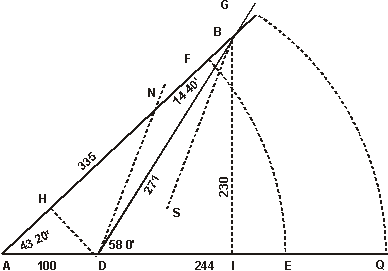The First Book of the Cross-Staff

CHAPT. IV The use of Lines of equal parts joyned with the Lines of Chords.

The Lines of equal parts do serve also for protraction, as may appear by the former Diagrams, but being joyned with the Lines of Chords, which I place upon one side of the Cross, they will further serve for the protraction and resolution of right Lined Triangles; whereof I will give one example in finding a distance at two stations otherwise than in the Second Chapter.

Let the distance required be AB, at A the first station, I make choice of a station Line towards C, and observe the Angle BAC by the Tangent Lines, which may be 43 gr. 20 m. then having gone an hundred paces toward C, I make my second station at D, where suppose I find the Angle BDC to be 58 gr. or the Angle BDA to be 112 gr. this being done, I may find the distance AB in this manner.

1. I draw a right Line AC, refresenting the station Line.
2. I take 100 out of the Lines of equal parts, and prick them down from A the first station unto D the second.
3. I open my Compasses to one of the Cords of 60 gr. and setting one foot on the point A, with the other I describe an occult Ark of a Circle intersecting the station Line in E.
4. I take out of the same Line of Chords a Chord of 43 gr. 20 m. (because such was the Angle at the first station) and this I inscribe into that occult Ark from E unto F, which makes the Angle FAD equal to the Angle observed at the first station.
5. I descibe another like Ark upon the Center D, and inscribe into it a Chord of 53 gr. from C unto G, and draw the right Line DG, which doth meet with the other Line AF in the Point B, and makes the Angle BDC equal to the Angle observed at the second station. So the Angles in the Diagram being equal to the Angles in the field, their sides will also proportional; and therefore,
6. I take out Line AB with my Compasses, and measuring it in the same Line of equal parts, from which I took AD, I find it to be 335, and such is the distance required.Zurück zum Inhaltsverzeichnis Nächstes Kapitel© Rainer Stumpe URL: www.rainerstumpe.de